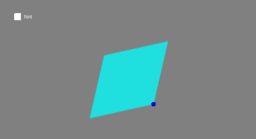# Posing Problems w/ Interactive Images II:reflective teaching

This GeoGebra book is the principal online resource for the second course in a two semester sequence on posing K-12 mathematics problems. The courses are designed for middle and secondary school teachers in the "Mathematics for Teaching" graduate program at the Harvard Extension School. The primary goal of this course is to give teachers a more systematic and theoretical framework for formulating the mathematics problems they pose to their students. Many (although not all) of the mathematics problems that we pose to K-12 students in order to stimulate invention and creativity can be classified along several several pairs of perspectives. These pairs of perspectives seem to overlap in some cases and in some cases to contrast with one another. In this course we explore some of these perspectives and the degree to which a sensitivity to them can help teachers pose problems both in class and for homework. The aim is to help teachers help their students to develop deeper insight into, and appreciation for, mathematics. In the course teachers work on problems that stimulate their inventiveness and creativity and then analyze the approaches both they and their colleagues have taken. The course relies heavily on interactive images, computer applets that use multiple graphical representations of the mathematical problems. One perspective can be described as follows: - the mathematical situation described by the problem presents a phenomenon that must be decomposed into component representations and analyzed to be understood - the mathematical situation described by the problem presents phenomena that must be combined into an aggregated representation to be understood Another perspective is one that characterizes problems on the basis of whether the resolution of the problem calls for analysis (deduction) or synthesis (induction). Classifying and exemplifying form the basis of yet another perspective. This perspective is well-known by teachers although probably not as widely used as it should be. It is the contrast between - Given a specific mathematical object and asking “What is this mathematical object a case of?” and, - Given a set of attributes and asking to generate (one, many, all) the mathematical object(s) that possess this set of attributes. Another perspective is one of Universal and Existential quantification. This rather formal perspective is increasingly present as students progress through their mathematical education. In some respects it echoes elements common to all of these perspectives. But these issues are also present in informal settings. The words we use in common parlance in building models of spatially distributed phenomena (All over, Somewhere, Nowhere) or temporally evolving events (All the time, Sometimes, Never) reflect the essence of Universal and Existential quantification. Finally, Polya in his seminal book on mathematics education “Mathematical Discovery” distinguishes two categories of problems – problems to prove and problems to find. [This distinction is fully discussed in Chapter V of Volume 1]. Students will be asked to explore the degree to which Polya’s distinctions map onto the other perspectives explored in the course (and vice versa). Case studies drawn from introductory geometry, algebra and arithmetic and their application will be analyzed in detail. Suggested background readings include the following essays on the mathMINDhabits website https://sites.google.com/site/mathmindhabits/- "What Makes A Good Challenge ?" "On Problem Posing & Making Conjectures"" "Formulating Measures" "Chicken Is To Data As Model Is To Egg" Some comments on the organization of these materials The interactive images, or applets in this collection(1) are organized in “matrix” form – there are three “ROWS” (algebra, arithmetic, geometry} and three “COLUMNS” {Decomposition & Aggregation, Induction & Deduction, Classification & Exemplification} Decomposing / aggregating Deductive / inductive approaches Classifying / exemplifying Algebra 1 4 7 Arithmetic 2 5 8 Geometry 3 6 9 Users of the materials are urged to examine each interactive image or applet not only by its particular COLUMN coordinate but by the appropriateness of each of the other columns as a perspective for understanding the applet and its usefulness in helping to pose problems. Users are also urged to explore how the links among the algebra, arithmetic and geometry ROWS might permit them to pose problems that span {algebra & arithmetic}, {arithmetic & geometry}, and {geometry & algebra}. (1) The applets in this collection are drawn from the "mathMINDhabits" archive https://sites.google.com/site/mathmindhabits/